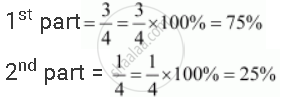Share

# Convert Each Part of the Ratio to Percentage: 3:1 - CBSE Class 7 - Mathematics

ConceptRatios to Percents

#### Question

Convert each part of the ratio to percentage:

3:1

#### Solution

3: 1

Total parts = 3 + 1 = 4Is there an error in this question or solution?

#### APPEARS IN

NCERT Solution for Mathematics for Class 7 (2018 to Current)
Chapter 8: Comparing Quantities
Ex. 8.30 | Q: 2.1 | Page no. 171
Solution Convert Each Part of the Ratio to Percentage: 3:1 Concept: Ratios to Percents.
S